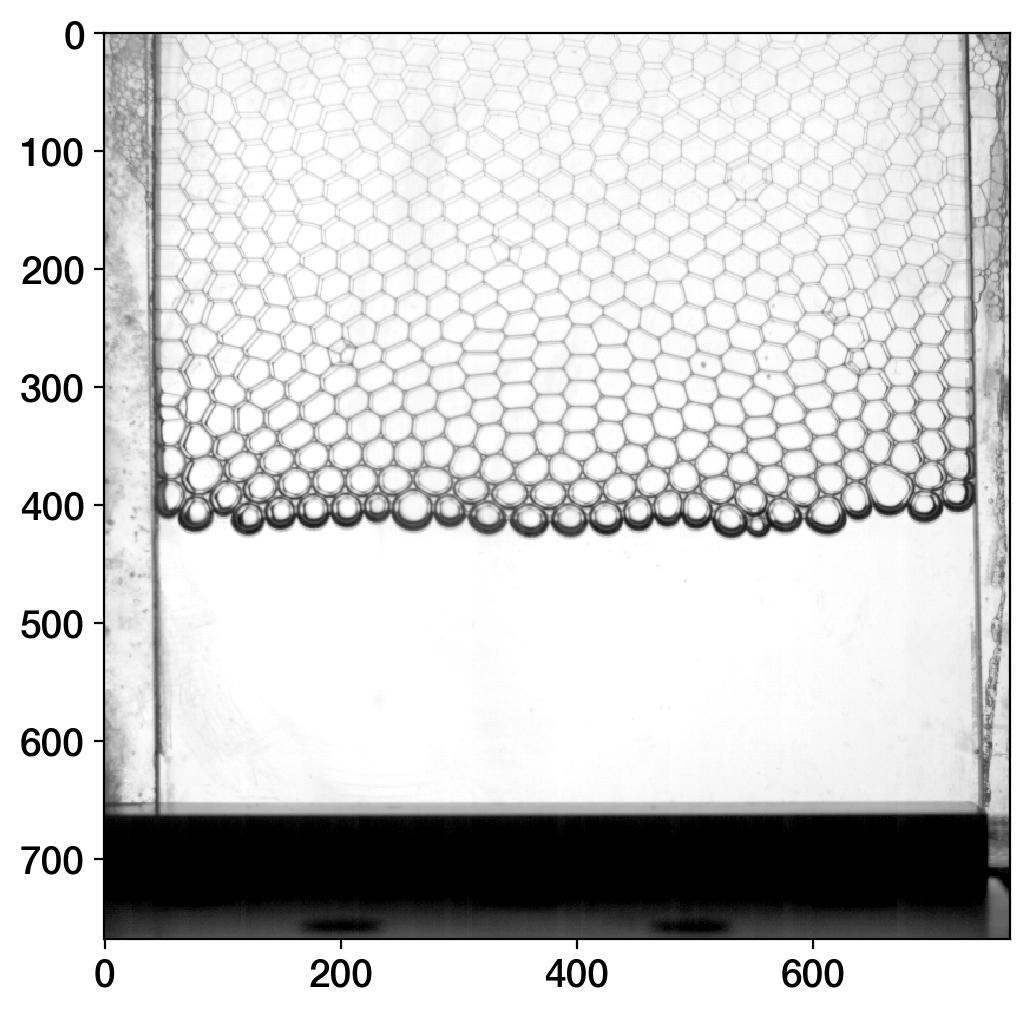# Custom Feature Detection and Velocity Fields: Bubble tracking in 2D foams¶

As illustrated in the walktrough example, the tracking relies on two steps:

• the detection of features
• the tracking of these features

In many situations, we track point-like particles. However, it is also possible to track extended features such as bubbles. Trackpy provides a 'locate' function to detect particles, seen as spots. But, in the case of bubbles in foams, bubbles are in contact with their neighbors and the 'locate' function could not detect their position.

In this notebook, we show that we can apply a custom method to detect the position of the bubbles and then, pass the list to the tracking algorithm.

## Scientific libraries¶

In :
# change the following to %matplotlib notebook for interactive plotting
%matplotlib inline

import numpy as np
import pandas as pd

import pims
import trackpy as tp
import os

import matplotlib  as mpl
import matplotlib.pyplot as plt

# Optionally, tweak styles.
mpl.rc('figure',  figsize=(10, 6))
mpl.rc('image', cmap='gray')

In :
datapath = '../sample_data/foam'
prefix = 'V1.75f3.125000'


## Image preprocessing¶

First, we load the batch of pictures and we display the first image

In :
id_example = 4
rawframes = pims.open(os.path.join(datapath, prefix + '*.tif'))
plt.imshow(rawframes[id_example]);Because, we will need to apply several image processing steps to detect the positions of the bubbles, we crop the picture to keep only the region of interest. With PIMS, this is done by defining a pipeline function to process the frames.

In :
@pims.pipeline
def crop(img):
"""
Crop the image to select the region of interest
"""
x_min = 45
x_max = -35
y_min = 100
y_max = -300
return img[y_min:y_max,x_min:x_max]

rawframes = crop(pims.open(os.path.join(datapath, prefix + '*.tif')))
plt.imshow(rawframes[id_example]);